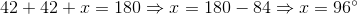# ISEE Upper Level Math : How to find an angle in an acute / obtuse isosceles triangle

## Example Questions

### Example Question #3 : Isosceles Triangles

One of the base angles of an isosceles triangle is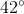. Give the measure of the vertex angle.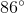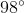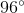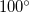The base angles of an isosceles triangle are always equal. Therefore both base angles are.
Let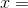the measure of the third angle. Since the sum of the angles of a triangle is, we can solve accordingly: